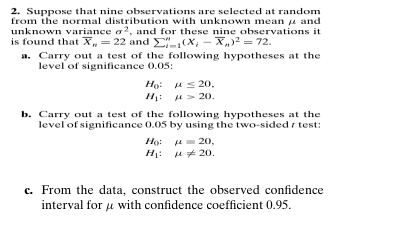# Question 2. Suppose that nine observations are selected at random from the normal distribution with unknown mean $$\mu$$ and unknown variance $$\sigma^{2}$$, and for these nine observations it is found that $$\bar{X}_{n}=22$$ and $$\sum_{i=1}^{n}\left(X_{i}-\bar{X}_{n}\right)^{2}=72$$.a. Carry out a test of the following hypotheses at the level of significance $$0.05$$ :$\begin{array}{ll}H_{0}: & \mu \leq 20 . \\H_{1}: & \mu \leq 20 .\end{array}$b. Carry out a test of the following hypotheses at the level of significance $$0.05$$ by using the two-sided $$t$$ test:$\begin{array}{ll}H_{0}: & \mu=20, \\H_{1}: & \mu \neq 20 .\end{array}$c. From the data, construct the observed confidence interval for $$\mu$$ with confidence coefficient $$0.95 .$$JH1UIC The Asker · Probability and Statistics
2. Suppose that nine observations are selected at random from the normal distribution with unknown mean $$\mu$$ and unknown variance $$\sigma^{2}$$, and for these nine observations it is found that $$\bar{X}_{n}=22$$ and $$\sum_{i=1}^{n}\left(X_{i}-\bar{X}_{n}\right)^{2}=72$$.
a. Carry out a test of the following hypotheses at the level of significance $$0.05$$ :
$\begin{array}{ll} H_{0}: & \mu \leq 20 . \\ H_{1}: & \mu \leq 20 . \end{array}$
b. Carry out a test of the following hypotheses at the level of significance $$0.05$$ by using the two-sided $$t$$ test:
$\begin{array}{ll} H_{0}: & \mu=20, \\ H_{1}: & \mu \neq 20 . \end{array}$
c. From the data, construct the observed confidence interval for $$\mu$$ with confidence coefficient $$0.95 .$$Transcribed Image Text: 2. Suppose that nine observations are selected at random from the normal distribution with unknown mean $$\mu$$ and unknown variance $$\sigma^{2}$$, and for these nine observations it is found that $$\bar{X}_{n}=22$$ and $$\sum_{i=1}^{n}\left(X_{i}-\bar{X}_{n}\right)^{2}=72$$. a. Carry out a test of the following hypotheses at the level of significance $$0.05$$ : $\begin{array}{ll} H_{0}: & \mu \leq 20 . \\ H_{1}: & \mu \leq 20 . \end{array}$ b. Carry out a test of the following hypotheses at the level of significance $$0.05$$ by using the two-sided $$t$$ test: $\begin{array}{ll} H_{0}: & \mu=20, \\ H_{1}: & \mu \neq 20 . \end{array}$ c. From the data, construct the observed confidence interval for $$\mu$$ with confidence coefficient $$0.95 .$$
More
Transcribed Image Text: 2. Suppose that nine observations are selected at random from the normal distribution with unknown mean $$\mu$$ and unknown variance $$\sigma^{2}$$, and for these nine observations it is found that $$\bar{X}_{n}=22$$ and $$\sum_{i=1}^{n}\left(X_{i}-\bar{X}_{n}\right)^{2}=72$$. a. Carry out a test of the following hypotheses at the level of significance $$0.05$$ : $\begin{array}{ll} H_{0}: & \mu \leq 20 . \\ H_{1}: & \mu \leq 20 . \end{array}$ b. Carry out a test of the following hypotheses at the level of significance $$0.05$$ by using the two-sided $$t$$ test: $\begin{array}{ll} H_{0}: & \mu=20, \\ H_{1}: & \mu \neq 20 . \end{array}$ c. From the data, construct the observed confidence interval for $$\mu$$ with confidence coefficient $$0.95 .$$